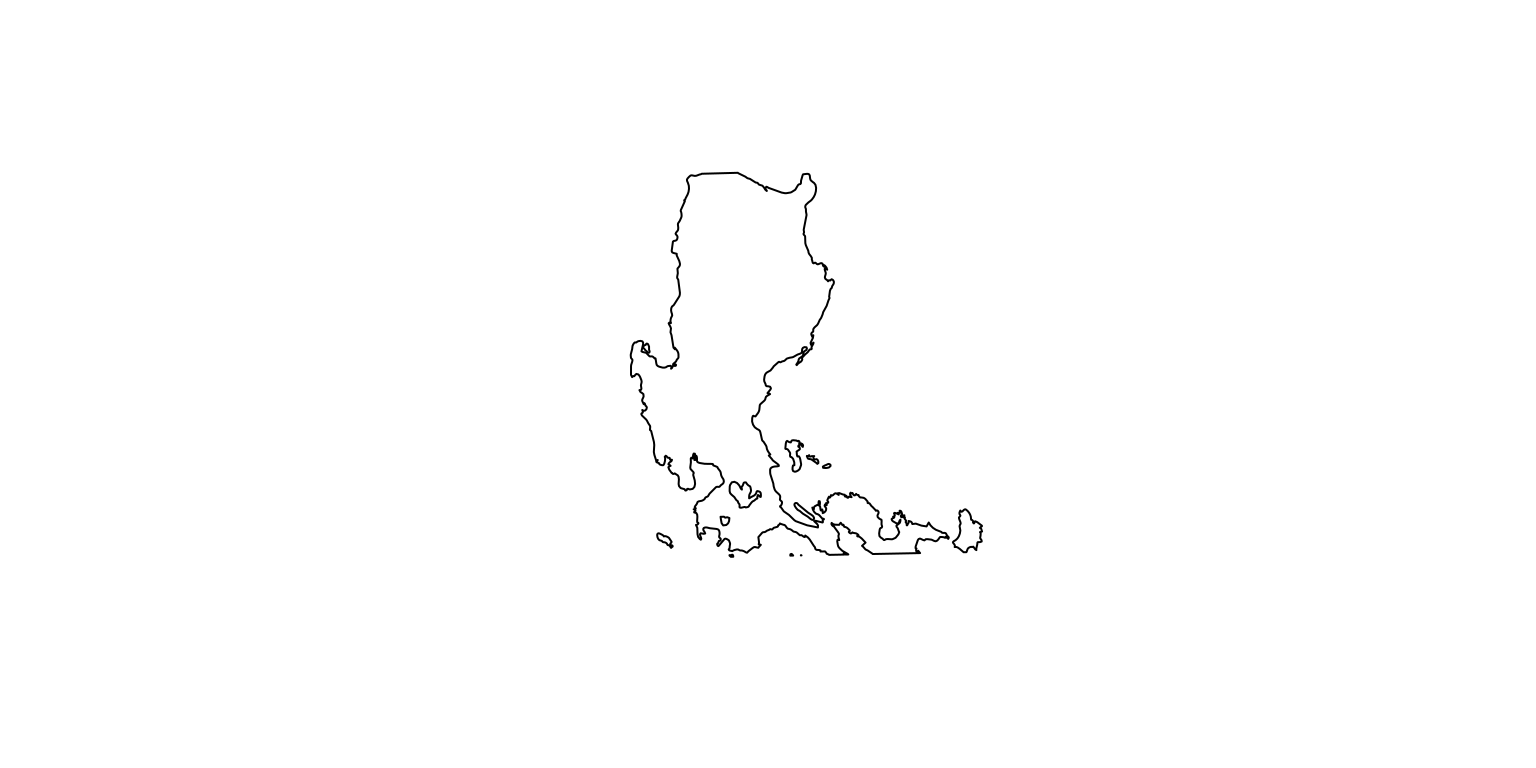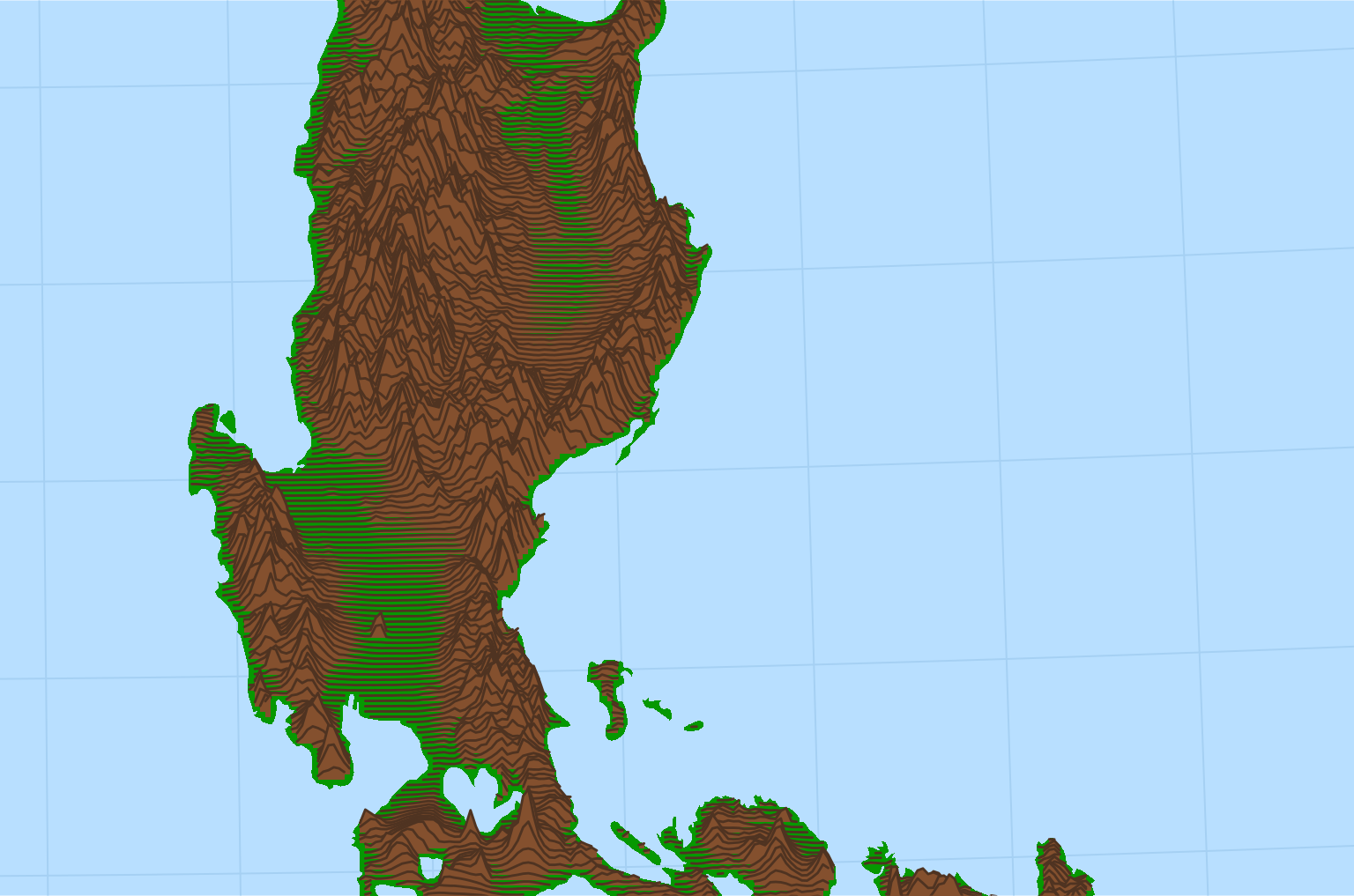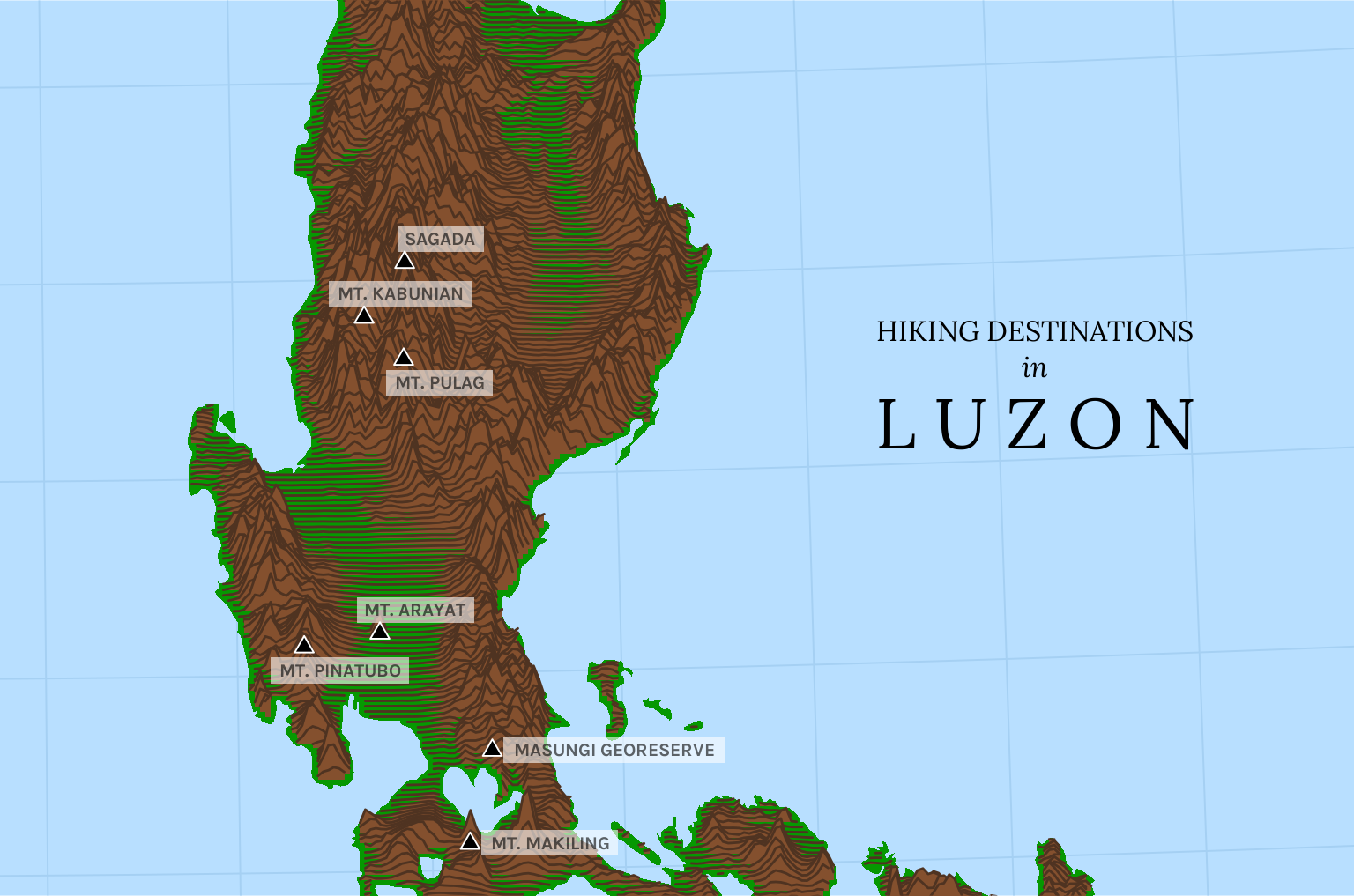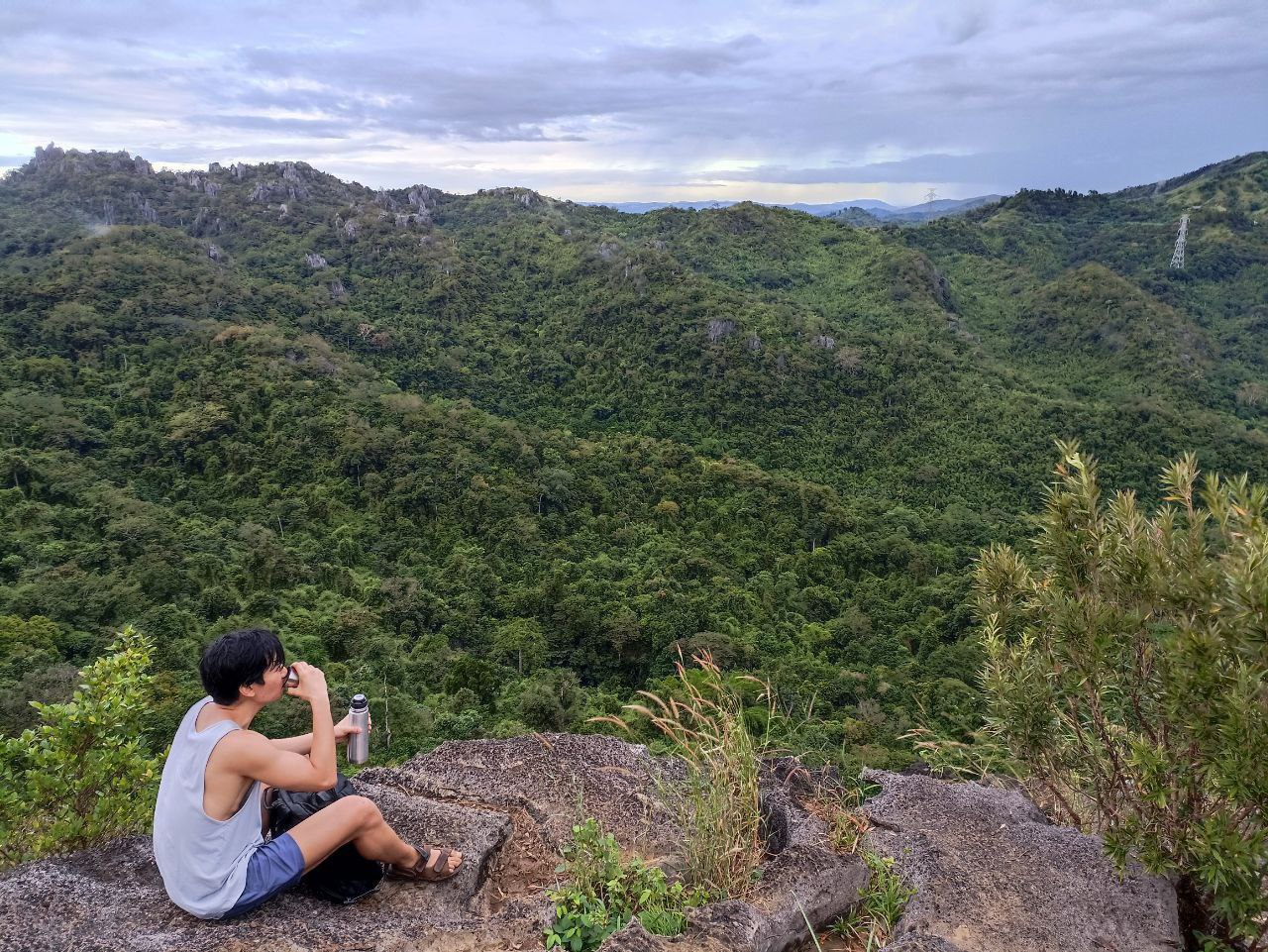# Luzon’s hiking trails

Creating a stylized map of mountains using ridgelines

R
ggplot
Published

December 15, 2022

I have lately been experimenting with `R`’s map-making capabilities, and as a project I wanted to try visualizing the great mountain ranges of Luzon. The Philippines has some remarkable mountain ranges, and hiking through them is a unique sort of pleasure that I only discovered recently.

An obvious approach would be to make a relief map, with colors corresponding to elevation, but to make it more interesting I decided to create a more stylized ridgeline-style map. A ridge plot is… hard to describe. Look up the album cover of Joy Division’s Unknown Pleasures and you’ll understand. I am indebted to dieghernan’s tutorial on mapping with ridgelines.1

``````library(tidyverse)
library(sf)
library(terra)``````

The coastline of the Philippines can be obtained from the `rnaturalearth` package.

``````library(rnaturalearth)
library(rnaturalearthdata)
library(rnaturalearthhires)

ph <- ne_countries(scale = 10, country = "Philippines", returnclass = "sf")

luzon <- ph %>%
st_crop(
xmin = st_bbox(ph)\$xmin %>% as.numeric(),
xmax = st_bbox(ph)\$xmax %>% as.numeric(),
ymin = 13.5,
ymax = 18.5
) %>%
st_transform(25391)``````

This returns a simple features or `sf` object, which has a `geometry` column that draws the coastline of the Philippines. The `st_crop()` function subsets the polygon to our area of interest while the `st_transform()` function sets the coordinate reference system to “Luzon 1911 / Philippines zone I” (EPSG code 25391).

Because this only draws the coastline, the major Luzon lakes of Laguna de Bay and Taal are not defined. We can load their polygons from `rnaturalearth` as well:

``````lakes <- ne_download(scale = 10, type = "lakes", category = "physical", returnclass = "sf") %>%
mutate(in_luzon = str_detect(name, "Laguna de Bay|Taal")) %>%
filter(in_luzon == TRUE) %>%
st_transform(25391) %>%
summarize()``````

We can then use `lakes` to “punch holes” into the `luzon` polygon:

``````luzon <- st_difference(st_geometry(luzon), st_geometry(lakes))

plot(luzon)``````To draw the ridges that symbolize Luzon’s mountain ranges, we need elevation raster data. This is taken from the `elevatr` package using the `get_elev_raster()` function.

``````library(elevatr)

dem <- get_elev_raster(luzon, z = 7, clip = "bbox", expand = NULL) %>%
rast() %>%

names(dem) <- "elev"``````

The `rast()` function converts it into a `SpatRaster` object, which is native to the `terra` package. The `mask()` function removes all cells outside the `luzon` polygon.

This raster actually provides more granularity than we want, so we aggregate the cells up to get a lower resolution. Then we convert it to a `data.frame` for plotting with `ggplot2`.

``````dem_agg <- aggregate(dem, round(nrow(dem) / 200))
dem_df <- as.data.frame(dem_agg, xy = TRUE, na.rm = FALSE)``````

The `luzon` polygon is plotted using `geom_sf()` while the ridgelines obtained from elevated data are plotted using `geom_ridgeline()` from the `ggridges` packages.

``````library(ggplot2)
library(ggridges)

# Set expanded boundaries
coords <- st_bbox(c(xmin = 118.75, xmax = 125.75, ymin = 13.75, ymax = 18.25),
crs = 4326
) %>%
st_as_sfc() %>%
st_transform(25391) %>%
st_coordinates()

map <- ggplot() +
geom_sf(data = luzon, color = NA, fill = "#069801") +
geom_ridgeline(
data = dem_df,
aes(x = x, y = y, group = y, height = elev),
scale = 25, fill = "#84502e", color = "#4f3321"
) +
coord_sf(
xlim = coords[c(1, 2), 1],
ylim = coords[c(2, 3), 2],
expand = FALSE
) +
scale_x_continuous(expand = c(0, 0)) +
scale_y_continuous(expand = c(0, 0)) +
theme_ridges() +
theme(
plot.margin = margin(0, 0, 0, 0),
panel.background = element_rect(fill = "#b8dfff"),
panel.grid.major = element_line(color = "#a5d0f3"),
axis.title = element_blank(),
axis.text = element_blank(),
axis.ticks.length = unit(0, "cm"),
)
map``````You can see how vividly the ridgelines capture Luzon’s mountains. It’s practically 3D!

I now want to add markers for some of the more famous hiking trails in Luzon. I can easily get their coordinates using Google Maps, but a complication to deal with is that the coordinate reference system (CRS) of Google Maps is different from the one I’m using here. I must therefore use `st_transform()` to reproject them first.

``````spots <- here::here("datasets", "hikingspots.csv") %>%
mutate(label = toupper(label))

coords <- spots %>%
st_as_sf(agr = "constant", coords = c("x", "y"), crs = 4326) %>%
st_transform(25391) %>%
st_coordinates()

spots <- cbind(spots, coords)``````

Code
``````library(ggrepel)

map +
geom_point(
data = spots,
aes(x = X, y = Y),
size = 2.5, shape = 24, linewidth = .75, color = "white", fill = "black"
) +
geom_label_repel(
data = spots,
aes(x = X, y = Y, label = label),
family = "karla", fontface = "bold", size = 8 / .pt,
hjust = .5, vjust = .5, nudge_x = 10000, min.segment.length = 1, label.r = unit(0, "lines"), label.size = NA, label.padding = unit(.2, "lines"), alpha = .6
) +

# Fancy plot title
annotate("text",
x = 1.27 * 10^6, y = 1.855 * 10^6, hjust = .5,
label = "HIKING DESTINATIONS", family = "lora", size = 12 / .pt
) +
annotate("text",
x = 1.27 * 10^6, y = 1.835 * 10^6, hjust = .5,
label = "in", family = "lora", fontface = "italic", size = 12 / .pt
) +
annotate("text",
x = 1.27 * 10^6, y = 1.803 * 10^6, hjust = .5,
label = "L U Z O N", family = "lora", size = 30 / .pt
)``````My one hiking experience so far was at Nagpatong Rock, near Masungi. It was arduous and muddy and I slept for 12 hours afterwards. But for that brief moment at the peak, as I sipped cold coffee and braced myself against the wind, I looked out at the view and found it absolutely grand.Stay elevated!## Footnotes

1. And let me just say that learning to make maps in `R` has been bewilderingly difficult. I have found Geocomputation with R useful, but much of it remains mysterious to me.↩︎